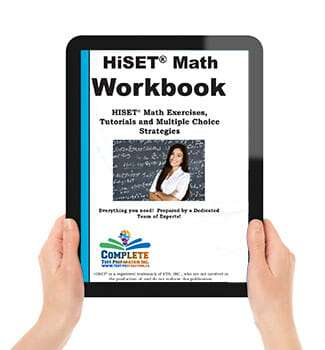## Taking the HiSET®? Need some extra math practice ?

### Coordinate Geometry

Slope of a Line
Parallel and perpendicular lines

### Practice

Pythagorean Geometry Tutorials, Examples and Practice Questions
Area of complex Shapes – Tutorial and Examples
Basic Geometry (Parallel lines, Angles, Distance and more)
Properties of Triangles
Calculating Slope

### Pre-Algebra

Basic Math
Simple Statistics
Interpreting Data
Probability

### Practice

Basic Math – 10 questions
Basic Math 2 – 10 questions
Word Problems Tutorial
How to Solve Word Problems
Percent – 18 questions
Fractions, Percent and Decimals – 14 questions
Scientific Notation – Complete Tutorial and 6 questions
Order of Operation – 6 questions
Square Root

Basic Statistics
Probability
Making inferences from a sample
Means, Median and Mode

Linear Equations
Exponents

### Intermediate Algebra

Linear equations with 2 variables
Functions
Polynomials
Sequences

### Need to brush up on your math? This is the book for you!

HiSET® Math practice questions, easy-to-read tutorials explaining everything in plain language, exam tips and tricks, math shortcuts, and multiple choice strategies! Everything you need, compiled by a dedicated team of experts with everything you need all in one place!

### Here is what the HiSET® Math Workbook can do for you:

• Learn then practice your math skills! Practice test questions are the best way to prepare for an exam and this is the book that you need to fully prepare for the HiSET® math test.
• Practice Tests familiarize you with the exam format and types of questions, giving you more confidence when you take the exam.
• Practice tests are a critical self-assessment tool that reveals your strengths and weaknesses.
• Practice tests allow you to practice your exam time management – a critical exam-writing skill that can easily improve your grade substantially.
• Practice tests reduce Test Anxiety, one of the main reasons for low marks on an exam. Hundreds of questions with detailed solutions and explanations to improve your understand of the basic concepts behind the questions.
• Learn powerful multiple choice strategies designed by exam experts! Includes tips and multiple choice strategies to increase your score you won’t find anywhere else!

### Heard it all before?

Maybe you have heard this kind of thing before, and don’t feel you need it. Maybe you are not sure if you are going to buy this book.

Remember though, it only a few percentage points divide the PASS from the FAIL students!

Even if our test tips increase your score by a few percentage points, isn’t that worth it?

## Over 200 HiSET® Practice Questions, Tutorials, Test Tips, and Multiple Choice Strategies!Over 200 HiSET® Math practice questions, plus test tips, how to study math, multiple choice strategies and more!

Order NOWPages: 206
Langugage: English

Written by,

Date Published: Friday, June 19th, 2020
Date Modified: Monday, July 27th, 2020

### HiSET PracticeHiSET ® Practice Test Questions - Includes math, social studies, science, English, plus how to write and essay and more.

Try a FREE Quiz

Getting Started
The HiSET® Study Plan 9
Making a Study Schedule 10

Basic Math
Fraction Tips, Tricks and Shortcuts 16
Converting Fractions to Decimals 18
Converting Fractions to Percent 20
Decimal Tips, Tricks and Shortcuts 22
Converting Decimals to Fractions 22
Converting Decimals to Percent 22
Percent Tips, Tricks and Shortcuts 23
Converting Percents to Decimals 24
Converting Percents to Fractions 25
Scientific Notation 25
How to Convert to Scientific Notation 26
Exponents Tips, Shortcuts & Tricks 28
Basic Math Practice 32

Word Problems
How to Solve Word Problems 52
Types of Word Problems 55
Word Practice 70

Basic Geometry
Cartesian Plane and Coordinate Grid 86
Pythagorean Geometry 92
Geometry Practice Questions 105

Basic Algebra
Solving One-Variable Linear Equations 141
Solving Two-Variable Linear Equations 142
Simplifying Polynomials 144
Factoring Polynomials 144
Algebra Practice Questions 151

Trigonometry 190
Sequences 193
Logarithms 194

Basic Math Multiple Choice
Multiple Choice Strategy and Shortcuts 228

How to Study for a Math Test

How to Prepare for a Test
The Strategy of Studying 236
How to Take a Test